WEBINAR
Magnetic Levitation for Energy Harvesting
Thursday, June 22, 2023
Time
SESSION 1
SESSION 2
CEST (GMT +2)
03:00 PM
08:00 PM
EDT (GMT -4)
09:00 AM
02:00 PM
HOME / Applications / Trimmed Resistor

# Trimmed Resistor

Used Tools:## Definition

Laser trimming is the manufacturing process of using a laser to adjust the operating parameters of an electronic circuit.

One of the most common applications uses a laser to burn away small portions of resistors, raising their resistance value. The burning operation can be conducted while the circuit is being tested by automatic test equipment, leading to optimum final values for the resistor in the circuit (Figure 1).

The resistance value of a film resistor is defined by its geometric dimensions (length, width, height) and the resistor material. A lateral cut in the resistor material by the laser narrows or lengthens the current flow path and increases the resistance value. The same effect is obtained whether the laser changes a thick-film or a thin-film resistor on a ceramic substrate or an SMD-resistor on a SMD circuit. The SMD-resistor is produced with the same technology and may be laser trimmed as well.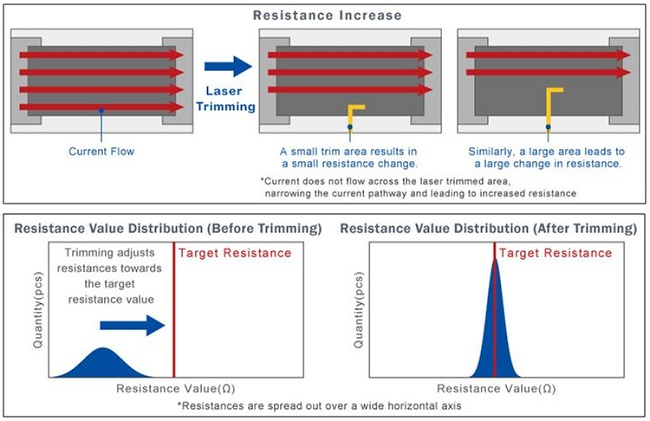Figure 1 - Laser trimming process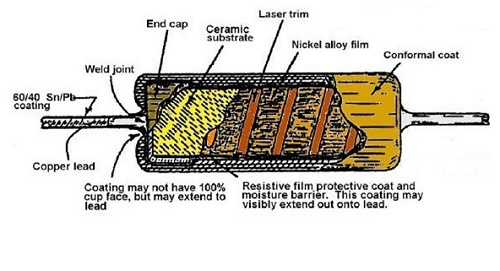Figure 2 - Resistor cut-away

## Description

Given a trimmed resistor made of copper and backed up by an aluminium slab subject to a voltage load of 10 volts applied at the left and right extremities.  The aim of this study is to compute the resistance as well as the electric field, current density, and the potential distributions.  Note that for the Electric Conduction analysis no air shall be modeled.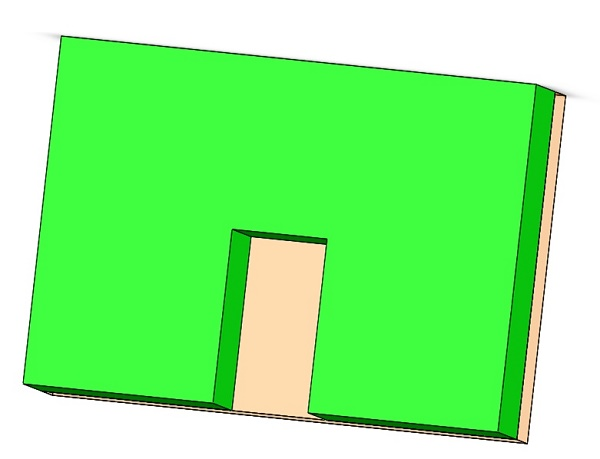Figure 3 - 3D model of trimmed resistor

## Study

The Electric Conduction is the only module that may compute the resistance. The resistance is defined as the ratio of voltage to current. The voltage is defined between two points. Therefore, the user has to specify the entry and exit ports for the resistor set. The entry port is where the current flows into the resistor set and exit port is where the current exits. EMS automatically computes the voltage difference between the entry and exit ports as well as the current flowing through the resistor set.  From the current and voltage, the resistance is deduced.
After creating a Electric Conduction study  in EMS, four important steps shall always be followed: 1 - apply the proper material for all solid bodies, 2- apply the necessary boundary conditions, or the so called Loads/Restraints in EMS, 3 - mesh the entire model and 4- run the solver.

## Materials

In the Electric Conduction analysis of EMS, only electric and thermal conductivity (in case of thermal coupling) are needed (Table 1).

Table1 - Table of materials

 Components / Bodies Material Conductivity (S/m) Trimmed Aluminum 38.2e+006 Part 2 Gold 41e+006

In this study, fixed voltage (Table 2) and resistance sets (Figure 4) are applied.

Table 2 - Applied Fixed Voltage

 Name Fixed Voltage Front Electrode 0.6 V Back Electrode 0 V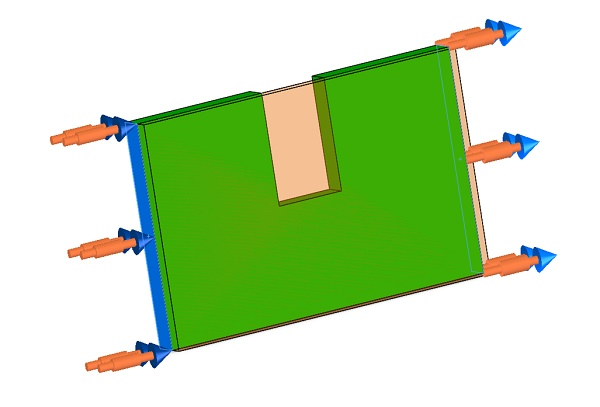Figure 4 - Resistance Sets applied in the body where we want to calculate the resistance

## Meshing

Meshing is a very crucial step in the design analysis. EMS estimates a global element size for the model taking into consideration its volume, surface area, and other geometric details. The size of the generated mesh (number of nodes and elements) depends on the geometry and dimensions of the model, element size, mesh tolerance, and mesh control. In the early stages of design analysis where approximate results may suffice, you can specify a larger element size for a faster solution. For a more accurate solution, a smaller element size may be required.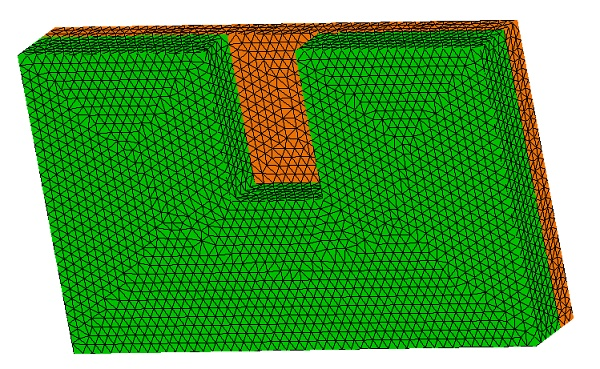Figure 5 - Meshed Model

## Results

After running the simulation of this example we can obtain many results. Electric Conduction of EMS is used to compute and visualize Electric Field (Figure 7,8), Current Density (Figure 9,10) and potential(Figure 11). A results table (Figure 6) also generated contains the resistance and dissipated power.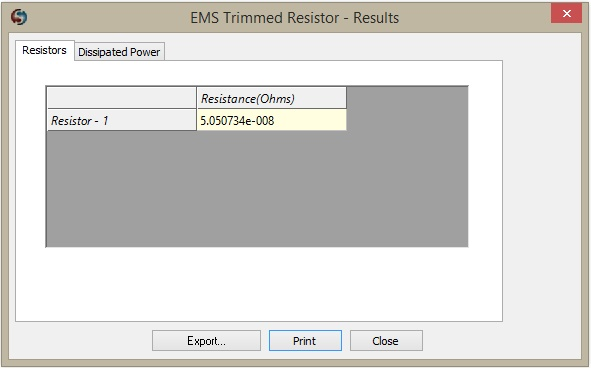Figure 6 - Results Table

EMS offers the possibility of many types of plot. Below we can observe fringe and vector plot of the magnetic flux density.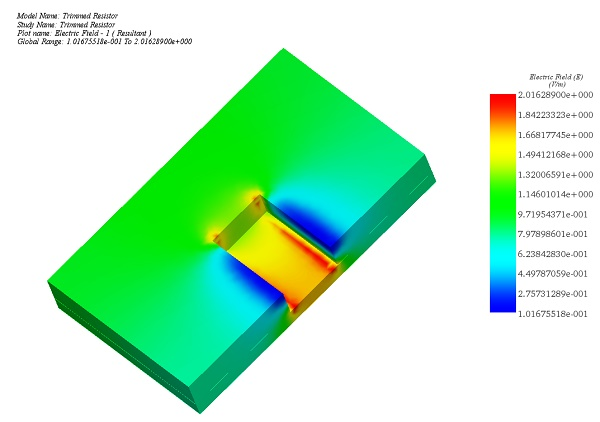Figure 7 - Electric Field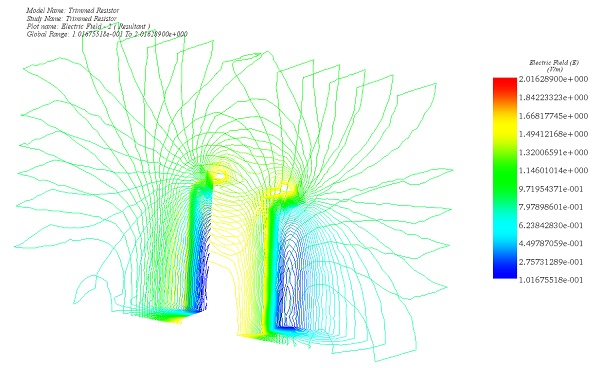Figure 8 - Electric Field, line plot (Iso clipping)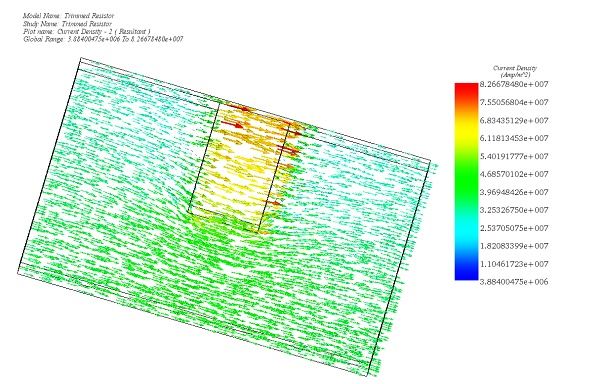Figure 9 - Current Density, vector plot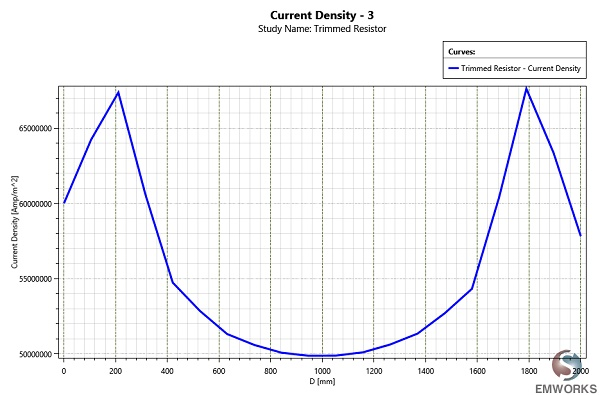Figure 10 - Line segment plot of Current Density between two points in the opposite faces of trim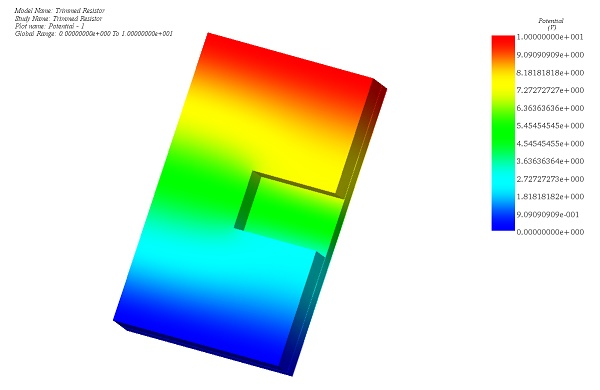Figure 11 - Potential

## Conclusion

Laser trimming of resistors allows integrated circuit manufacturers to very accurately control resistance values. Being an expensive process step, it is important to maximize the benefits once that option is taken. EMS Electric Conduction module allows easy characterization of different trim algorithms.